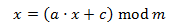class template
<random>

# std::linear_congruential_engine

`template <class UIntType, UIntType a, UIntType c, UIntType m>class linear_congruential_engine;`
Linear congruential random number engine
A pseudo-random number generator engine that produces unsigned integer numbers.

This is the simplest generator engine in the standard library. Its state is a single integer value, with the following transition algorithm:Where x is the current state value, a and c are their respective template parameters, and m is its respective template parameter if this is greater than 0, or numerics_limits<UIntType>::max() plus 1, otherwise.

Its generation algorithm is a direct copy of the state value.

This makes it an extremely efficient generator in terms of processing and memory consumption, but producing numbers with varying degrees of serial correlation, depending on the specific parameters used.

The random numbers generated by linear_congruential_engine have a period of m.

### Template parameters

UIntType
An unsigned integer type.
Values produced by the engine are of this type.
a
The multiplier parameter (a) used in the transition algorithm.
If m is not zero, this parameter should be lower than m.
c
The increment parameter (c) used in the transition algorithm.
If m is not zero, this parameter should be lower than m.
m
The modulus parameter (m) used in the transition algorithm, except if this parameter is zero.
If this parameter is zero, the value assumed for m on all operations is numerics_limits<UIntType>::max() plus one (even if UIntType cannot represent this value).

### Member types

The following alias is a member type of list_congruential_engine:

member typedefinitionnotes
result_typeThe first template parameter (UIntType)The type of the numbers generated.

### Member constexpr constants

member constantdefinitionnotes
multiplierThe second template parameter (a)The multiplier (a) used in the transition algorithm on each advance.
incrementThe third template parameter (c)The increment (c) used in the transition algorithm on each advance.
modulusThe fourth template parameter (m)The modulus (m) used in the transition algorithm on each advance.
default_seed1uThe default seed used on construction or seeding.